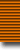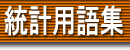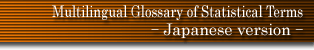### 用語検索

and or
検索単位 単語 フレーズ 完全一致 〜で始まる 〜を含む 〜で終わる 日本語 英語 ドイツ語 フランス語
 日本語 英語 ドイツ語 フランス語
 radico-Normal distributions radix Radon-Nikodym theorem Raikov's theorem raising factor random random allocation design random axis random balance design random bifurcation random component random distribution random effects model random error random event random impulse process random linear graph random Normal scores test random order random orthogonal transformation random process random sample random sampling error random sampling numbers random selection random series random start random tesselation random variable random walk randomisation randomisation tests randomised blocks randomised decision function randomised fractional factorial designs randomised model randomised response randomised significance tests randomised test range range chart rank rank correlation rank order statistics rank scores rank transformation rankit rank-randomisation tests Rao-Blackwell theorem Rao-Kupper model Rao's scoring test ratio ratio delay method ratio estimator ratio scale rational trend raw moment raw score Rayleigh distribution Rayleigh tests realisation reciprocal scaling record linkage records tests recovery of information rectangular association scheme rectangular distribution rectangular lattice rectified index number rectifying inspection rectilinear trend recurrence game recurrence time recurrent Markov chain recurrent state recursive algorithm recursive system reduced design reduced equations reduced form method reduced inspection reduced sample reduced variate reduction of data Reed-Frost model Reed-Münch method reference period reference set reflecting barrier refusal rate regenerative process region of indifference region of preference regressand regression regression coefficient regression curve regression dependence regression diagnostics regression estimate regression line regression surface regression weight regressogram regressor regret regular best asymptotically normal estimator regular estimator regular group divisible incomplete block design regular Markov renewal process regular state regular stationary point process reification rejectable quality level rejection error rejection line rejection number rejection region rejective sampling relative area of transvariation relative efficiency (of a sample design) relative efficiency (of a test) relative efficiency (of an estimator) relative frequency relative index relative information relative potency relative precision relative risk relative variance relaxed oscillation reliability reliability coefficient relvariance remote sensing renewal density renewal distribution renewal function renewal process renewal theorem renewal theory repeated measures design repeated survey repetition repetitive group sampling plan replacement replacement process replication representative sample reproducibility resemblance residual residual effect residual sum of squares residual treatment effect residual variance residual waiting time resistant techniques resolvable balanced incomplete block design resolvable designs response response error response metameter response surface response surface designs response time distribution R-estimator restricted chi-square test restricted maximum likelihood restricted randomisation restricted sequential procedure retrospective study return period return states return time reversal design reversal test reversible relation Rhodes' distribution Rice distribution ridge regression ridit analysis Riemann distribution right and wrong cases method right angular design Ripley's statistics risk risk function Robbins-Munro process robust estimator robustness Room's squares root estimator (of mean) root mean square deviation root mean square error rose diagram Rosenbaum's test rotatable design rotation rotation sampling roughness penalty Round Robin design rounding route sampling R-technique ruin problems runs Rutherford's contagious distribution